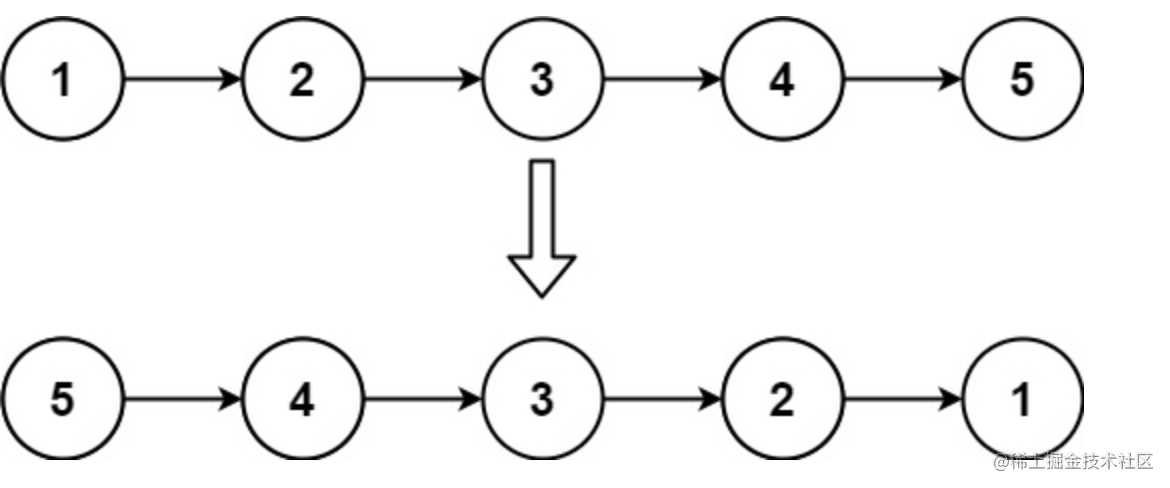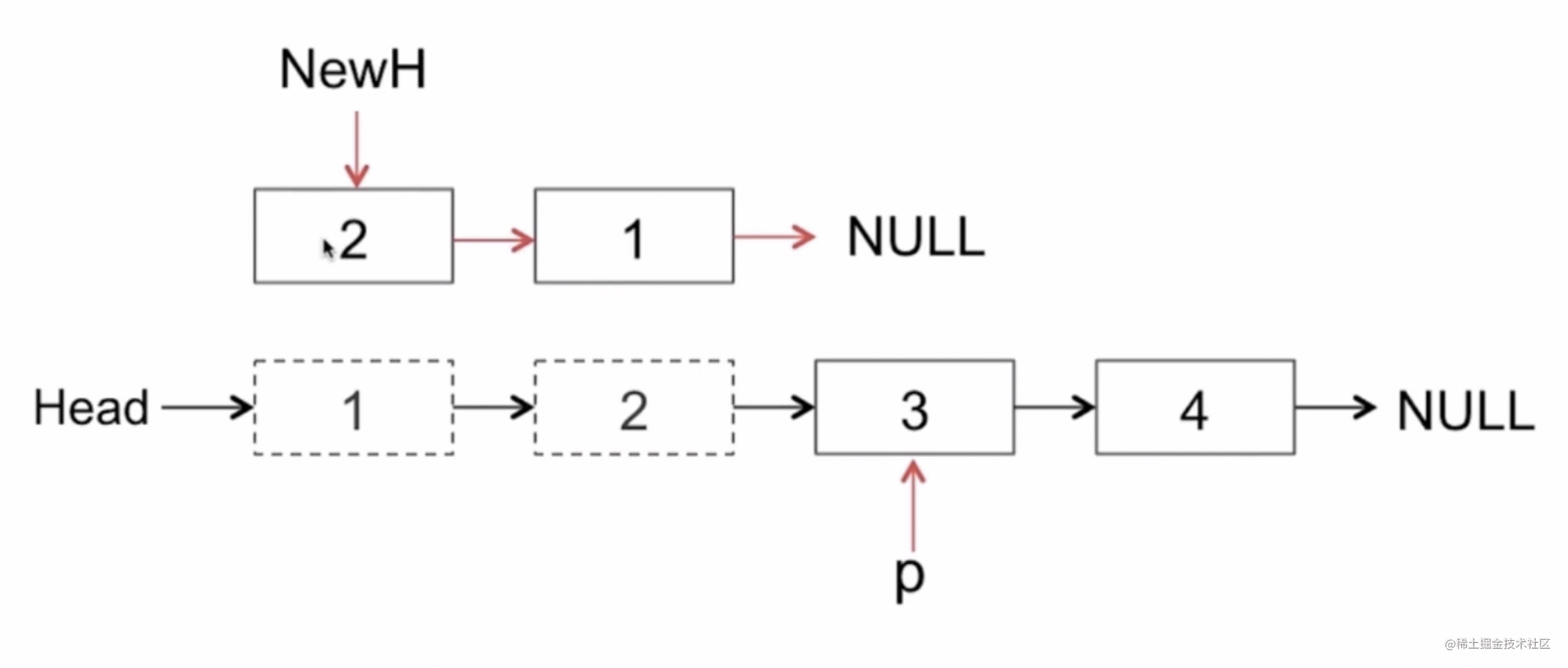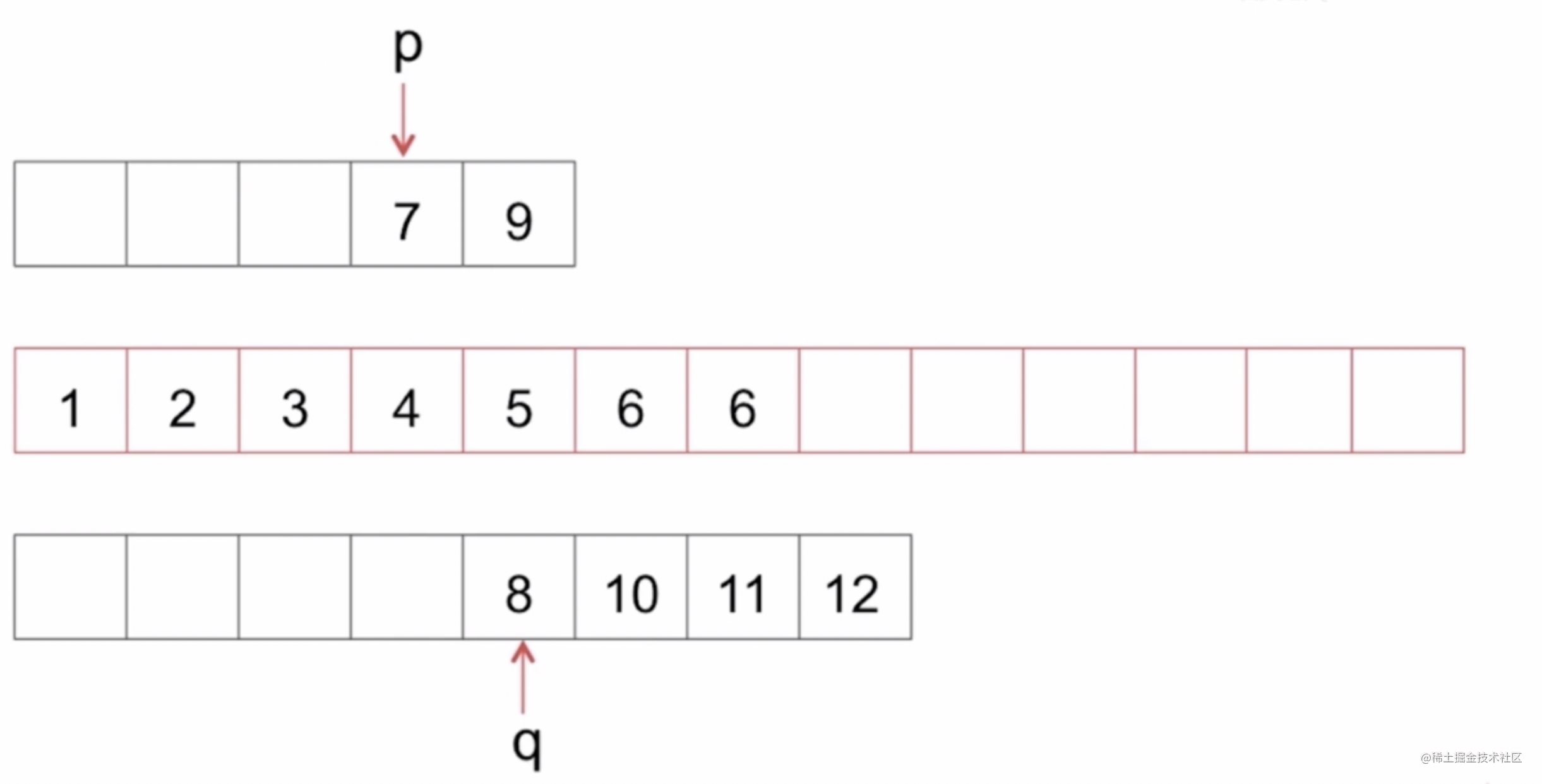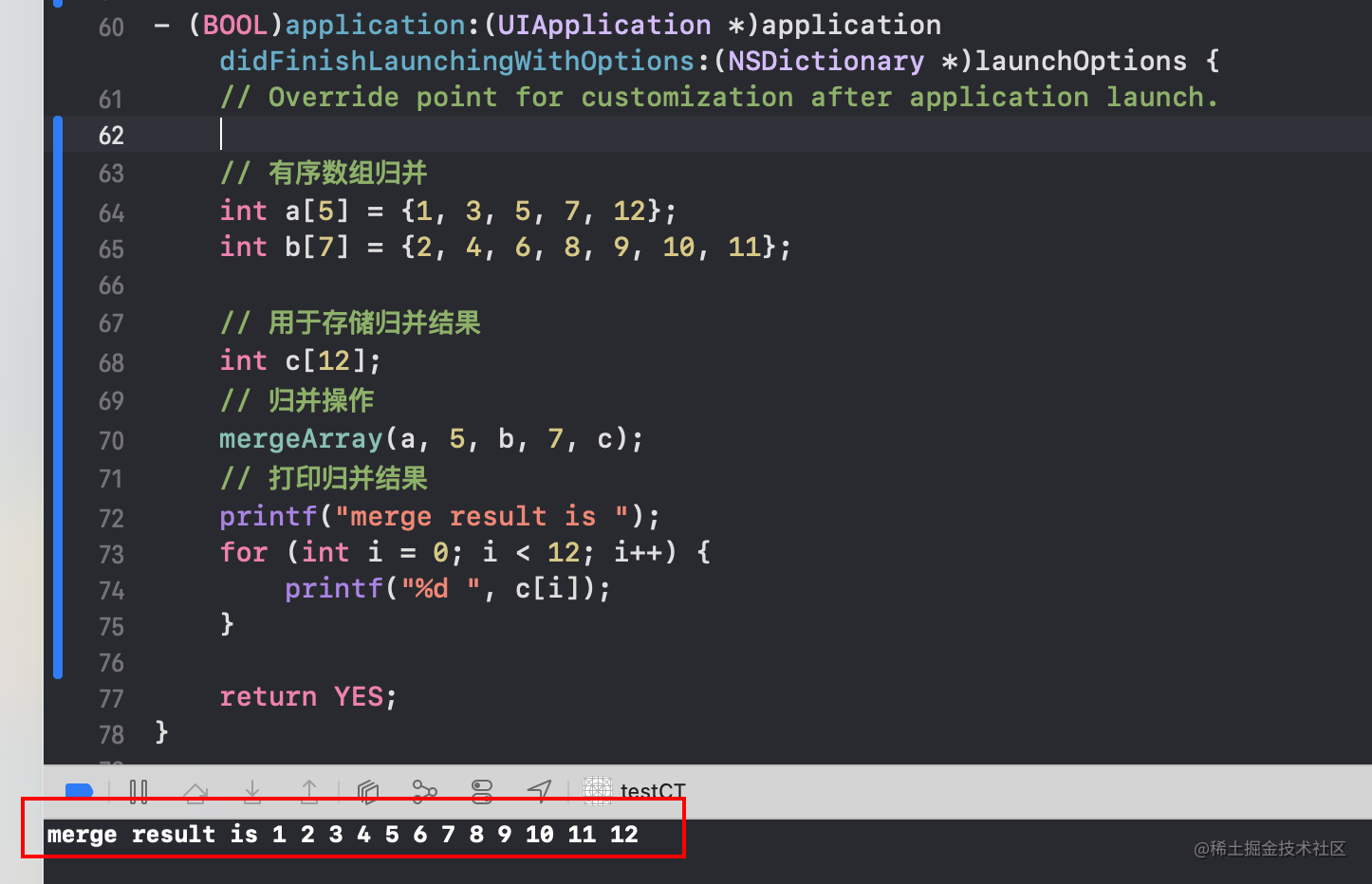# iOS老司機整理, iOSer必會的經典演算法_1

## 前言

• iOS日常開發中, 演算法使用的多嗎? 實事求是的來說, 是不多的.
• 那演算法的學習對iOSer來說, 就不需要了嗎? 答案是很需要.
• iOS的日常開發中, 用到的演算法確實不多, 但是演算法在iOS開發裡面的應用都被封裝在各種常用API的內部了.
• 對演算法的學習, 可以提高對iOS底層的理解與認識, 這些演算法的知識是計算機技術領域通用的. 下面就iOS開發中一些經典的演算法進行一個簡單的梳理.
• 文章純手打, 拋磚引玉, 如有錯誤還請評論區指正, 先行謝過了:)

# 1. 字串反轉

• 編寫一個函式，其作用是將輸入的字串反轉過來。輸入字串以字元陣列 `s` 的形式給出。不要給另外的陣列分配額外的空間，你必須原地修改輸入陣列、使用 O(1) 的額外空間解決這一問題。

```輸入： s = ["h","e","l","l","o"] 輸出： ["o","l","l","e","h"]```

```輸入： s = ["H","a","n","n","a","h"] 輸出： ["h","a","n","n","a","H"]```

• 使用C語言的解答及思路: ```void reverseString(char* s, int sSize) { // 指向第一個字元 char *begin = s; // 指向最後一個字元 char *end = s + sSize - 1; while (begin < end) { // 交換前後兩個字元， 同時移動指標 char temp = *begin; *(begin++) = *end; *(end--) = temp; } }```
• 力扣連結

# 2. 單鏈表反轉

• 給你單鏈表的頭節點 `head` ，請你反轉連結串列，並返回反轉後的連結串列。如: ```輸入： head = [1,2,3,4,5] 輸出： [5,4,3,2,1]```• 採用頭插法的思路圖解:• 使用Swift語言的解答及思路: ``` /**
• public class ListNode {
• public var val: Int
• public var next: ListNode?
• public init() { self.val = 0; self.next = nil; }
• public init(_ val: Int) { self.val = val; self.next = nil; }
• public init(_ val: Int, _ next: ListNode?) { self.val = val; self.next = next; }
• } */

class Solution {

``````// 遞迴遍歷
// func reverseList(_ head: ListNode?) -> ListNode? {
//     }

// }

// 頭插法
func reverseList(_ head: ListNode?) -> ListNode? {
var prev : ListNode? = nil // 新建上一個結點點, 初始為nil
var current : ListNode? = head // 設定當前結點為傳入的結點

while current != nil {// while遍歷當前結點
let next : ListNode? = current?.next // 設定下一個結點為當前結點的next

current?.next = prev // 當前結點的next節點指向上一個結點
prev = current // 設定上一個結點為當前結點
current = next // 設定當前結點為下一個結點
}

return prev // 返回頭插的結點
}
``````

} ``` - 力扣連結

# 3. 有序數組合並

• 如何將有序陣列a和b的值合併到一個數組c當中, 且仍然保持有序?
• 演算法思路:
• 利用雙指標.• 使用C語言的解答及思路: ``` void mergeArray(int a[], int aLen, int b[], int bLen, int c[]) { // 遍歷陣列a的指標 int p = 0; // 遍歷陣列b的指標 int q = 0; // 記錄當前儲存位置 int i = 0;

// 任一陣列沒有到達邊界則進行遍歷 while (p < aLen && q < bLen) { // 如果a陣列對應位置的值小於b陣列對應位置的值 if (a[p] <= b[q]) { // 儲存a陣列的值 c[i] = a[p]; // 移動b陣列的遍歷指標 p++; } else { // 儲存b陣列的值 c[i] = b[q]; // 移動b陣列的遍歷指標 q++; }

``````//指向合併結果的下一個儲存位置
i++;
``````

}

// 如果a陣列有剩餘 while (p < aLen) { // 將a陣列剩餘部分拼接到合併結果的後面 c[i] = a[p++]; i++; }

// 如果b陣列有剩餘 while (q < bLen) { // 將b陣列剩餘部分拼接到合併結果的後面 c[i] = b[q++]; i++; } }

// 驗證程式碼: // 有序陣列歸併     int a = {1, 3, 5, 7, 12};     int b = {2, 4, 6, 8, 9, 10, 11};

// 用於儲存歸併結果     int c;     // 歸併操作     mergeArray(a, 5, b, 7, c);     // 列印歸併結果     printf("merge result is ");     for (int i = 0; i < 12; i++) {         printf("%d ", c[i]);     } ``` - 結果列印:#### 發文不易, 喜歡點讚的人更有好運氣👍 :), 定期更新+關注不迷路~

ps：歡迎加入筆者18年建立的研究iOS稽核及前沿技術的三千人扣群：662339934，坑位有限，備註“掘金網友”可被群管通過~